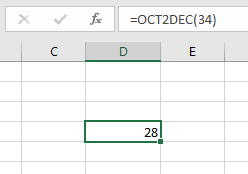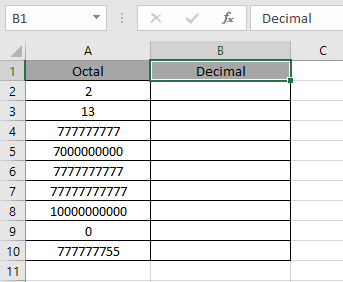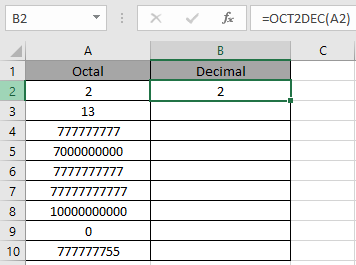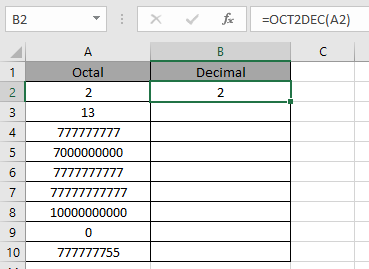# How to use the OCT2DEC Function in Excel

In this article, we will learn about how to use the OCT2DEC function in Excel.

OCT2DEC function in excel is used to convert octal representation of numbers of radix 8 to decimal numbers (radix = 2).

The table shown below shows you some of the most used base & their radix of Alpha - numeric characters

 Base radix Alpha-Numeric Characters Binary 2 0 - 1 Octal 8 0 - 7 Decimal 10 0 - 9 hexadecimal 16 0 - 9 & A - F hexatridecimal 36 0 - 9 & A - Z

decimal number is representation of a number of radix 10. 10 digits are used in representation of a decimal number 0, 1, 2, 3, 4, 5, 6, 7, 8 & 9. Where as octal number representation have only 8 digits from 0, 1, 2, 3, 4, 5, 6 & 7 The below table will help you understand better.

 Octal Decimal 0 0 1 1 2 2 3 3 4 4 5 5 6 6 7 7 10 8

Conversion formulation is shown below:
345

1. Separate the digits
2. Multiply the separated digit with 80, 81,82, 83 and so on starting from the rightmost digit correspondingly.
3. Get the sum of all the multiplied numbers. There it is our decimal number
 5 4 3 5 x 82 4 x 81 3 x 80 320 32 3 543 ( octal ) = 355( decimal )

The OCT2DEC function converts the octal number of radix 8 to the decimal number of radix 10.
Syntax:

=OCT2DEC ( number, [places] )

Number : Octal number which returns the 10 character decimal number.
The input value to the function cannot contain more than 10 characters ( 10 bits ). Where first number returns the sign of the number (positive or negative) & Other 9 returns the value after the sign. ( 1 for negative & 0 for positive).To get a negative number as output from the function, input number must have 10 characters starting with the one bit as it represents the negative sign. The negative number is calculated using two's complement notation. Any less than 10 characters, the function considers default 0s in front. Any more than 10 characters, the function returns the #NUM! Error.

Now let’s get more understanding of the function via using them in some examples.Here we have some octal values in Octal Column. We need to convert these octal numbers to decimal value.

Use the formula in octal column:

=OCT2DEC (A2)

A2 : number provided to the function as cell reference

Values to the OCT2DEC function is provided as cell reference.The OCT2DEC representation of 2 of base 8 (decimal) is 2 of base 10 (decimal).
Now copy the formula to other cells using the Ctrl + D shortcut key.As you can see here the OCT2DEC function returns the result of the input values.

Notes:

1. Numbers can be given as argument to the function directly without quotes or cell reference in excel.
2. Excel takes 7000000000 the lowest octal number converted to decimal value - 134217728
3. Excel takes 777777777  the highest octal number converted to decimal value  + 134217727
4. The function returns the #NUM! Error
1. If the input octal number is less than 7000000000 or greater than 777777777.
2. The input number must be valid octal number.
5. The function returns the #VALUE! Error
1. If the input number is text or non-numeric.

Hope you understood how to use OCT2DEC function and referring cell in Excel. Explore more articles on Excel mathematical conversion functions here. Please feel free to state your query or feedback for the above article.

Related Articles:

How to use the DEC2BIN Function in Excel

How to use the BIN2OCT Function in Excel

How to use the BIN2HEX Function in Excel

How to use the DEC2OCT Function in Excel

How to use the DEC2HEX Function in Excel

How to use the OCT2BIN Function in Excel

How to use the OCT2DEC Function in Excel

How to use the OCT2HEX Function in Excel

How to use the HEX2BIN Function in Excel

How to use the HEX2OCT Function in Excel

How to use the HEX2DEC Function in Excel

Popular Articles:

If with wildcards

Vlookup by date

Join first and last name in excel

Count cells which match either A or B

Terms and Conditions of use

The applications/code on this site are distributed as is and without warranties or liability. In no event shall the owner of the copyrights, or the authors of the applications/code be liable for any loss of profit, any problems or any damage resulting from the use or evaluation of the applications/code.